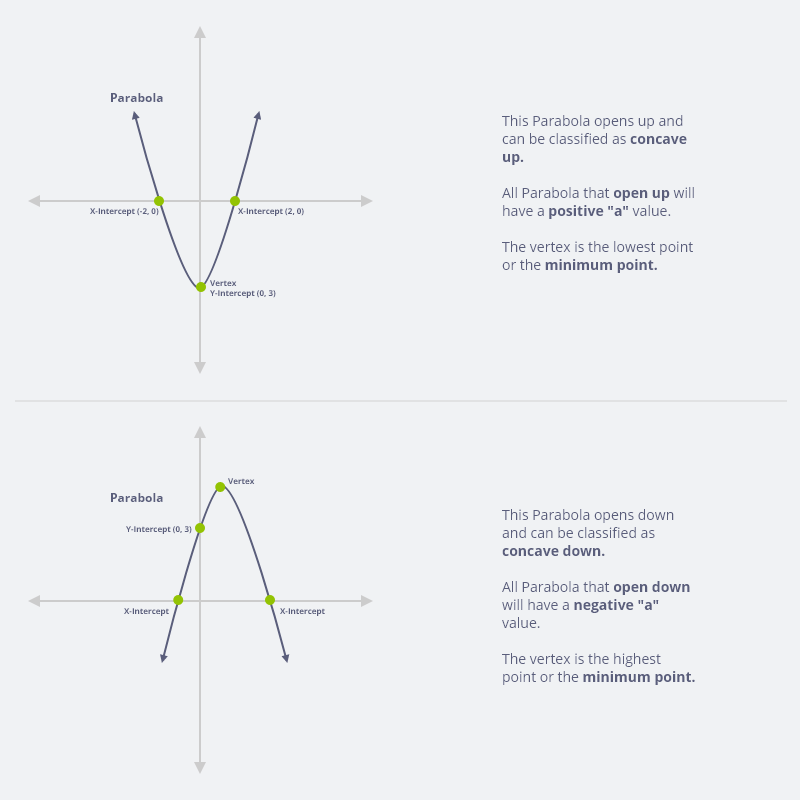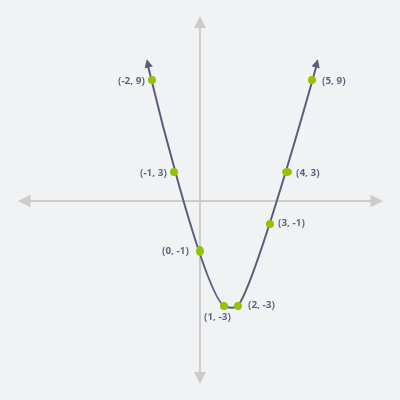### Introduction Of The Quadratic Equation:

The second-degree polynomial equation which has only one unknown variable is known as Quadratic equation. The standard form of Quadratic equation is as $$ax^2+ bx+c=0$$ and the formula for Quadratic equation is $$x = \dfrac{ -b \pm \sqrt{b^2 – 4ac}}{2a}$$. New inventions have made our work much easier just like Quadratic formula calculator which is the great Quadratic equation solver just put the values of a, b, & c and get your answer instantly.

### Methods Use To Solve The Quadratic Equation:

There are four methods to solve quadratic equations: Quadratic formula, completing the square method, factoring method, & the graphing. Amazing thing is that there are different Quadratic function calculators available online to solve quadratic equations in less time just like Quadratic formula calculator, & factoring quadratics calculator etc.

If you are not good at factoring and find the quadratic function calculator or find the best Quadratic equation solver then you are at the right place because our factoring quadratics calculator will solve your equations in minutes.

### Uses Of The Quadratic Equation:

The use of Quadratic equation is not only confined with mathematics, algebra, & geometry but in any field like chemistry, physics, daily science, and in accounting if we find any equation which has at least one square value or in Quadratic standard form then we may solve that equation by any of the above-mentioned methods. So, if you belong to any field and want to solve quadratic equations then you may also use Quadratic formula calculator or Quadratic function calculator without searching the formula for Quadratic equation.

### Graphing Or Sketch Parabola Of The Quadratic Equation:

If we solve quadratic equations graphically then it shows u shaped upwards and downwards and this u shaped is known as parabola. In Quadratic equation if the value of a is equal to 1 or greater than 1 then parabola opens upwards and if the value of a is less than 1 then parabola opens downwards as shown in the following:Let’s understand the graphing of the quadratic equation by an example:

### Example To Solve Quadratic Equation By Graph (Parabola):

Solve the quadratic equation by graph

$$y = x^2 – 3x – 1$$

Now we put the values of x to find the values of y:

If $$x = -2, y = (-2)^2 – 3 (-2) – 1 = 9$$

If $$x = -1, y = (-1)^2 – 3 (-1) – 1 = 3$$

If $$x = 0, y = (0)^2 – 3 (0) – 1 = – 1$$

If $$x = 1, y = (1)^2 – 3 (1) – 1 = – 3$$

If $$x = 2, y = (2)^2 – 3 (2) – 1 = – 3$$

If $$x = 3, y = (3)^2 – 3 (3) – 1 = – 1$$

If $$x = 4, y = (4)^2 – 3 (4) – 1 = 3$$

If $$x = 5, y = (5)^2 – 3 (5) – 1 = 9$$

$$X: -2, -1, 0, 1, 2, 3, 4, 5$$

$$Y: 9, 3, -1, -3, -3, -1, 3, 9$$In the above quadratic equation, we computed the coordinates for eight points. We noted that the plotting of any number of points for which x is less than 3 is not giving a shape of the curve and when the values of x are greater than 3 then it is giving a curve shape. Thus, it is important to choose the values of x for plotting the graph and generally we should determine at least five well-chosen values of x for getting an accurate sketch of a quadratic equation. We can see in the above graph that if the value of a is equal to 1 or a is positive then the parabola is opening upwards.

### Concepts That Are Related With The Graph Of Quadratic Equation:

Now we discuss the following concepts which are associated with the graph of Quadratic equation:

1. Roots.
2. Discriminant.
3. Vertex.
4. X-intercept.
5. Y-intercept.

#### Roots:

The Quadratic formula is also known as the roots of the quadratic equation and the formula for Quadratic equation is as:

$$x = \dfrac{ -b \pm \sqrt{b^2 – 4ac}}{2a}$$

We can write the roots of the quadratic equation as:

Root for $$x = \dfrac{ -b + \sqrt{b^2 – 4ac}}{2a}$$

Root for y $$x = \dfrac{ -b – \sqrt{b^2 – 4ac}}{2a}$$

#### Discriminant:

In Quadratic formula the part $$b^2 – 4ac$$ is known as Discriminant and it is represented by D and the nature of the roots of the quadratic equation depends on the value of the D.

If The Value Of The D = 0:

The roots of the quadratic equation will be equal.

If The Value Of The D Is Greater Than 0:

The roots of the quadratic equation will be real.

If The Value Of The D Is Less Than 0:

The roots of the quadratic equation will be imaginary.

If The Value Of The D Is Not A Perfect Square And Greater Than 0:

The roots of the quadratic equation will be irrational.

If The Value Of D Is A Perfect Square And Greater Than 0:

The roots of the quadratic equation will be rational.

If The Values Of A, B, & C Are Integers And The Value Of D Is Greater Than 0 And Also A Perfect Square:

The roots of the quadratic equation will be integers.

### Vertex:

In a Quadratic equation graph parabola, the maximum and the minimum point is known as vertex. We find the vertex of the quadratic equation by the following formulas:

Vertex for $$x = \dfrac{-b}{2a}$$

.

Vertex for $$y = \dfrac{-b^2 – 4ac}{4a}$$

.

We can also write the above vertex formulas as:

Vertex for $$x = h = \dfrac{-b}{2a}$$

.

Vertex for $$y = k = \dfrac{-D}{4a}$$

.

### X-Intercept:

In the quadratic equation if we want to find the x-intercept then we will put the value of y as 0 and will solve the equation simply by adding and subtracting.

### Y-Intercept:

Similarly, if we want to find out the y-intercept then we will put the value of the x as 0 and will solve the equation in a simple way.

So, if you are new to solving the quadratic equation graphically then from the above discussion you will be able to sketch the parabola of the Quadratic equation. In solving the quadratic equation if you are facing any problem and you are finding the quadratic equation solver and finding the formula for Quadratic equation or trying to solve quadratic equation by Quadratic function calculator then try our Quadratic formula calculator and get flying colors in your assignment.